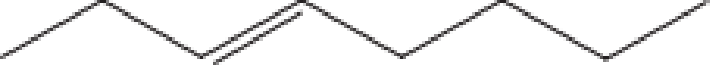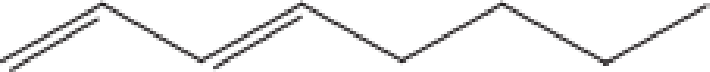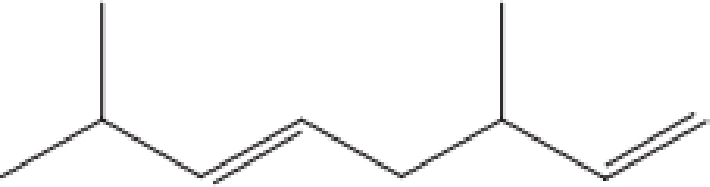Chapter 2, Problem 2.35EP### Organic And Biological Chemistry

7th Edition
STOKER + 1 other
ISBN: 9781305081079

#### Solutions

Chapter
Section### Organic And Biological Chemistry

7th Edition
STOKER + 1 other
ISBN: 9781305081079
Textbook Problem

# What is the IUPAC name for each of the compounds in Problem 13-31?a.b.c.d.(a)

Interpretation Introduction

Interpretation:

The IUPAC name for the given compound has to be written.

Concept Introduction:

IUPAC nomenclature for alkene:  There are about eight rules to be followed in giving IUPAC name for alkene.

• The suffix –ane has to be replaced with the suffix –ene.  This is used to indicate the presence of double bond.
• The longest continuous chain of carbon atoms has to be chosen that contains both carbon atoms of the double bond.
• The parent carbon chain has to be numbered in a way so that the numbering begins at the end near to the double bond.  In case if the double bond is equidistant from both ends, then numbering has to be done from the end that is closer to substituents.
• The position of the double bond has to be given a single number which is lower‑numbered carbon atom that is present in the double bond.
• Suffixes like –diene, -triene, -tetrene, and so on are used when the compound contains more than one double bond.
• In case of cycloalkenes which do not have any substitution, the numbering is not needed to locate the double bond because the bond is assumed to be between the carbons 1 and 2.
• In case if substituents are present in cycloalkene, then the double‑bonded carbon atoms are numbered 1 and 2 in a direction where the substituent gets the lower number.
• If the cycloalkenes contain more than one double bond, then one double bond is assigned the numbers 1 and 2 followed by the other double bond so that the lowest number possible is given.

Structural formula where a line represent carbon‑carbon bond and the carbon atom is considered to be present in each point and the end of lines is known as Line-angle structural formula.  To indicate a double bond between carbon atom, double line is used.

Explanation

Given compound is,

The longest continuous carbon chain present in the given compound is eight carbon atoms.  Hence, the parent alkane is octane.

As the given compound contains a double bond, the suffix –ane is replaced by –ene...

(b)

Interpretation Introduction

Interpretation:

The IUPAC name for the given compound has to be written.

Concept Introduction:

IUPAC nomenclature for alkene:  There are about eight rules to be followed in giving IUPAC name for alkene.

• The suffix –ane has to be replaced with the suffix –ene.  This is used to indicate the presence of double bond.
• The longest continuous chain of carbon atoms has to be chosen that contains both carbon atoms of the double bond.
• The parent carbon chain has to be numbered in a way so that the numbering begins at the end near to the double bond.  In case if the double bond is equidistant from both ends, then numbering has to be done from the end that is closer to substituents.
• The position of the double bond has to be given a single number which is lower‑numbered carbon atom that is present in the double bond.
• Suffixes like –diene, -triene, -tetrene, and so on are used when the compound contains more than one double bond.
• In case of cycloalkenes which do not have any substitution, the numbering is not needed to locate the double bond because the bond is assumed to be between the carbons 1 and 2.
• In case if substituents are present in cycloalkene, then the double‑bonded carbon atoms are numbered 1 and 2 in a direction where the substituent gets the lower number.
• If the cycloalkenes contain more than one double bond, then one double bond is assigned the numbers 1 and 2 followed by the other double bond so that the lowest number possible is given.

Structural formula where a line represent carbon‑carbon bond and the carbon atom is considered to be present in each point and the end of lines is known as Line-angle structural formula.  To indicate a double bond between carbon atom, double line is used.

(c)

Interpretation Introduction

Interpretation:

The IUPAC name for the given compound has to be written.

Concept Introduction:

IUPAC nomenclature for alkene:  There are about eight rules to be followed in giving IUPAC name for alkene.

• The suffix –ane has to be replaced with the suffix –ene.  This is used to indicate the presence of double bond.
• The longest continuous chain of carbon atoms has to be chosen that contains both carbon atoms of the double bond.
• The parent carbon chain has to be numbered in a way so that the numbering begins at the end near to the double bond.  In case if the double bond is equidistant from both ends, then numbering has to be done from the end that is closer to substituents.
• The position of the double bond has to be given a single number which is lower‑numbered carbon atom that is present in the double bond.
• Suffixes like –diene, -triene, -tetrene, and so on are used when the compound contains more than one double bond.
• In case of cycloalkenes which do not have any substitution, the numbering is not needed to locate the double bond because the bond is assumed to be between the carbons 1 and 2.
• In case if substituents are present in cycloalkene, then the double‑bonded carbon atoms are numbered 1 and 2 in a direction where the substituent gets the lower number.
• If the cycloalkenes contain more than one double bond, then one double bond is assigned the numbers 1 and 2 followed by the other double bond so that the lowest number possible is given.

Structural formula where a line represent carbon‑carbon bond and the carbon atom is considered to be present in each point and the end of lines is known as Line-angle structural formula.  To indicate a double bond between carbon atom, double line is used.

(d)

Interpretation Introduction

Interpretation:

The IUPAC name for the given compound has to be written.

Concept Introduction:

IUPAC nomenclature for alkene:  There are about eight rules to be followed in giving IUPAC name for alkene.

• The suffix –ane has to be replaced with the suffix –ene.  This is used to indicate the presence of double bond.
• The longest continuous chain of carbon atoms has to be chosen that contains both carbon atoms of the double bond.
• The parent carbon chain has to be numbered in a way so that the numbering begins at the end near to the double bond.  In case if the double bond is equidistant from both ends, then numbering has to be done from the end that is closer to substituents.
• The position of the double bond has to be given a single number which is lower‑numbered carbon atom that is present in the double bond.
• Suffixes like –diene, -triene, -tetrene, and so on are used when the compound contains more than one double bond.
• In case of cycloalkenes which do not have any substitution, the numbering is not needed to locate the double bond because the bond is assumed to be between the carbons 1 and 2.
• In case if substituents are present in cycloalkene, then the double‑bonded carbon atoms are numbered 1 and 2 in a direction where the substituent gets the lower number.
• If the cycloalkenes contain more than one double bond, then one double bond is assigned the numbers 1 and 2 followed by the other double bond so that the lowest number possible is given.

Structural formula where a line represent carbon‑carbon bond and the carbon atom is considered to be present in each point and the end of lines is known as Line-angle structural formula.  To indicate a double bond between carbon atom, double line is used.

### Still sussing out bartleby?

Check out a sample textbook solution.

See a sample solution

#### The Solution to Your Study Problems

Bartleby provides explanations to thousands of textbook problems written by our experts, many with advanced degrees!

Get Started